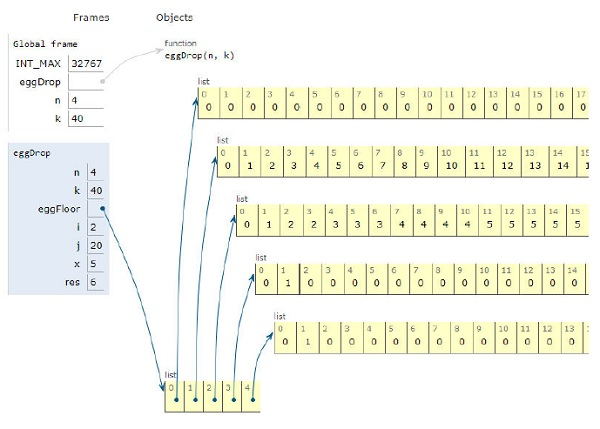# Python Program for Egg Dropping Puzzle

Problem statement − Suppose that we want to know which stories in a 40-story building are safe to drop the eggs from, and which of those will cause the eggs to get damaged on landing with the help of eggs. We need to display a minimum number of trails to check the stories.

Now let’s observe the solution in the implementation below −

## Example

Live Demo

# dynamic programming
INT_MAX = 32767
# to get minimum trials
def eggDrop(n, k):
# intialization
eggFloor = [[0 for x in range(k + 1)] for x in range(n + 1)]
# base case
for i in range(1, n + 1):
eggFloor[i] = 1
eggFloor[i] = 0
# We always need j trials
for j in range(1, k + 1):
eggFloor[j] = j
# Fill rest of the entries
for i in range(2, n + 1):
for j in range(2, k + 1):
eggFloor[i][j] = INT_MAX
for x in range(1, j + 1):
res = 1 + max(eggFloor[i-1][x-1], eggFloor[i][j-x])
if res < eggFloor[i][j]:
eggFloor[i][j] = res
return eggFloor[n][k]
# main
n = 4
k = 40
print("Minimum number of trials in worst case scenario with " + str(n) + " eggs and "+ str(k) + " floors is " + str(eggDrop(n, k)))

## Output

Minimum number of trials in worst case scenario with 4 eggs and 40 floors is 6All the variables are declared in the local scope and their references are seen in the figure above.

## Conclusion

In this article, we have learned about how we can make a Python Program for Egg Dropping Puzzle.